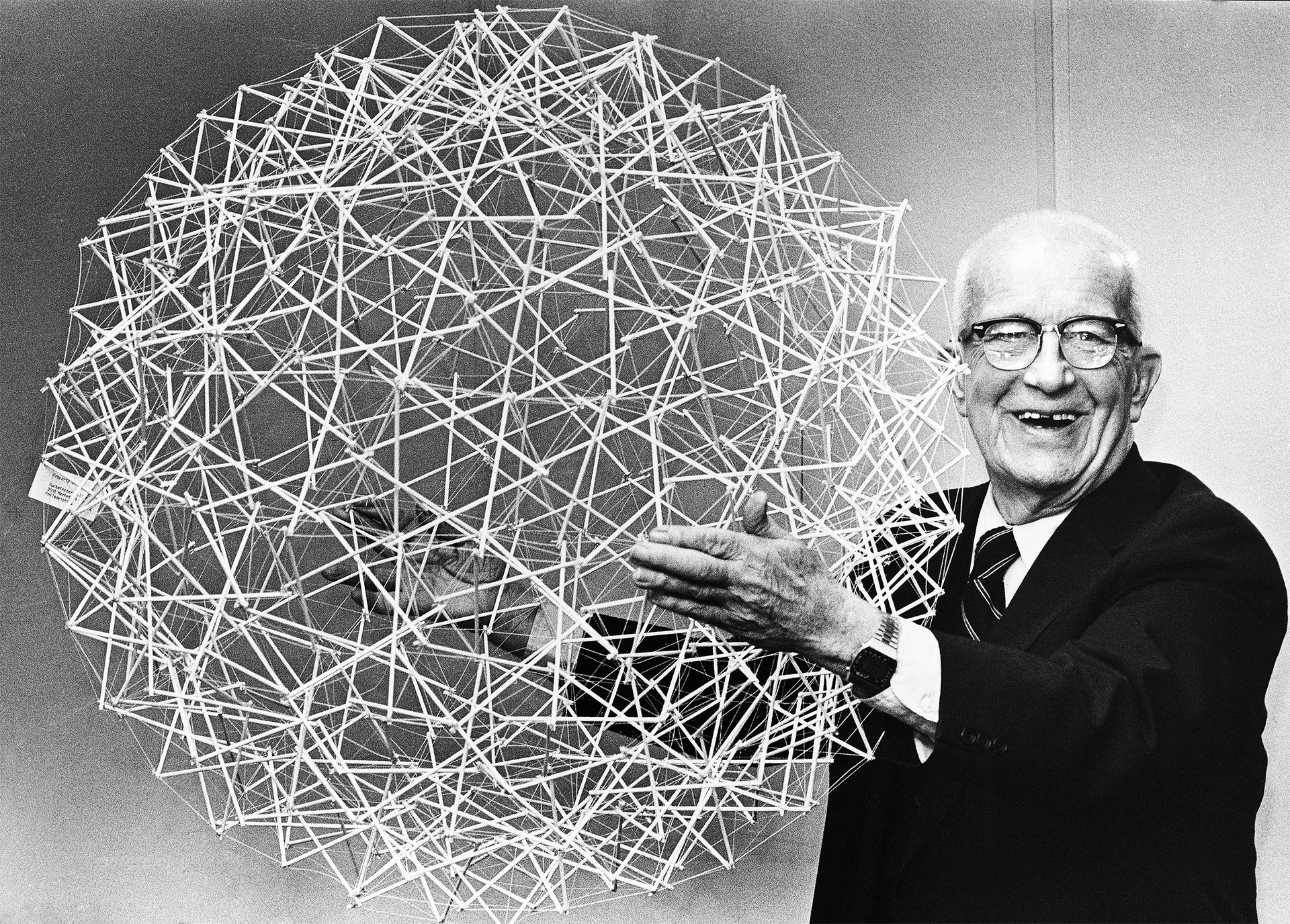# Lucky thirteen fits a mathematical formula

Lucky thirteen fits a mathematical formula. Thirteen is not such a bad number; as a matter of fact, it’s great.  Perhaps one of the greatest geniuses of all time fit 13 into a formula having to do with the packing of spheres: None other than Richard Buckminster Fuller. Fuller published more than 30 books, coining or popularizing such terms as “Spaceship Earth“, “Dymaxion” (e.g., Dymaxion houseDymaxion carDymaxion map), “ephemeralization“, “synergetics, and “tensegrity“.How many spheres can pack around a central sphere and then around those spheres in turn, etc.? The formula is the layer number of spheres squared, multiplied by ten and then add two.

The formula alluded to in the picture is  n² x 10 + 2. We start with one central sphere, then multiply it by ten and add two. Thus, 12 spheres can surround one central sphere. Next, go to the second layer. Formula becomes 2² x 10 + 2. Forty-two numbers the spheres in the second layer. The following happens:

• Twelve spheres can totally surround a central sphere of equal size. Counting the center, that makes 13.
• This central sphere and the twelve can then be totally encased by 42 in the next layer. That makes for a total of 55 spheres. etc.

### Lucky Thirteen Connotes a Complete and Balanced System

The above heading can also be true with an ancient system called gematria. In the Middle East, the same symbol could represent both a letter and number. Unity calls on number one in the Hebraic language. Letters look at the glyph for “one”:אֶחָד. Aleph stands for number one. Ches becomes the glyph for number eight. Dalet represents number four. Simply add the three numbers as 1 + 8 + 4 = 13. Here again, we find Fuller’s number.

How does this conclude? In unity there is peace. Diversity is okay, but only to a point. When everyone pulls in a different direction, most fall in the process. As a matter of fact, the most significant Hebraic prayer, the Shema Yisroel, declares the Oneness of the Creator.

Internal link: Periodic Chart Grid Found in the Lo Shu